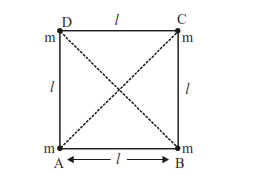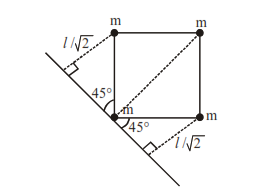Deepak Scored 45->99%ile with Bounce Back Crack Course. You can do it too!

# Four equal masses, m each are placed at the corners of a square of length (l) as shown in the figure.

Question:

Four equal masses, m each are placed at the corners of a square of length (l) as shown in the figure. The moment of inertia of the system about an axis passing through A and parallel to DB would be :1. $\mathrm{m} l^{2}$

2. $2 \mathrm{~m} l^{2}$

3. $3 \mathrm{~m} l^{2}$

4. $\sqrt{3} \mathrm{ml} l^{2}$

Correct Option: , 3

Solution:

Moment of inertia of point mass

$=$ mass $\times(\text { Perpendicular distance from axis })^{2}$Moment of Inertia

$=\mathrm{m}(0)^{2}+\mathrm{m}(l \sqrt{2})^{2}+\mathrm{m}\left(\frac{l}{\sqrt{2}}\right)^{2}+\mathrm{m}\left(\frac{l}{\sqrt{2}}\right)^{2}$

$=3 \mathrm{~m} l^{2}$